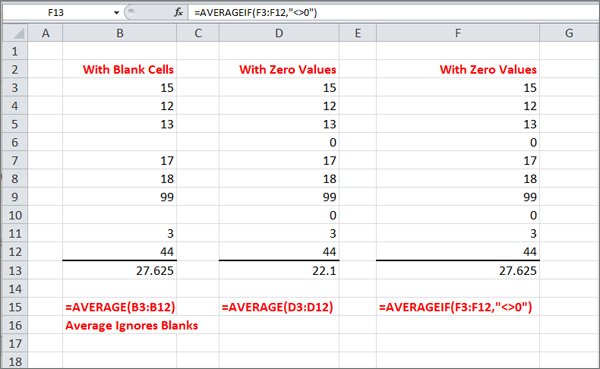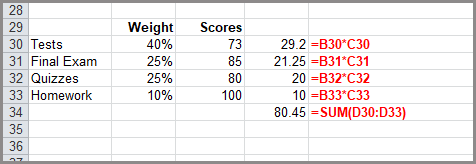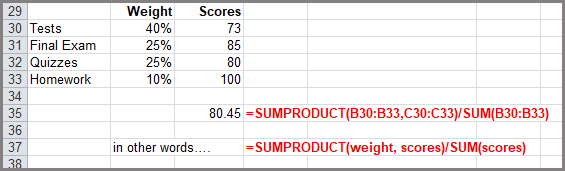Select Page

Share

### The Average() Function

The Excel AVERAGE function returns the average (arithmetic mean) of a range of values. It’s much like the SUM function where you enter the range of values you want summarized. For example A10:A50 or B3:B156.  The following example averages the values in the range B4:B9.### The AverageIF() Function

According to Microsoft, if a range or cell reference argument contains text, logical values, or empty cells, those values are ignored; however, cells with the value zero are included.

So if your range has zeros in it, instead of using =Average(), use =AverageIf().  In the following example, column B shows an average that includes blank cells.  In Column D, the same values are included but rather than blanks, some cells contain a zero.  Notice the difference in the way the average is calculated.  Column B adds up 8 numbers and divides the result by 8.  Column D adds up 8 numbers but divides the result by 10.

In column F, the AVERAGEIF function is told to only average the cells in the range that are not equal to zero.The syntax requires the range of cells and the criteria.

### Weighted Average

In the previous examples all of the numbers were given equal significance. The numbers were added together, and then, divided by the number of numbers. With a Weighted Average, one or more numbers is given a greater significance, or weight.

In the following example a student is enrolled in a course where the final grade is determined based on the following categories:

• Tests – 40%
• Final exam – 25%
• Quizzes – 25%
• Homework – 10%

The student has earned the following scores for each category:

• Tests – 73
• Final exam – 85
• Quizzes – 80
• Homework – 100.

We need to calculate the student’s overall grade.  Normally, you would multiply each weight by its score and then add those up.Or you can use the SUMPRODUCT and the SUM functions to find a Weighted Average, which depends on the weight applied to the values.